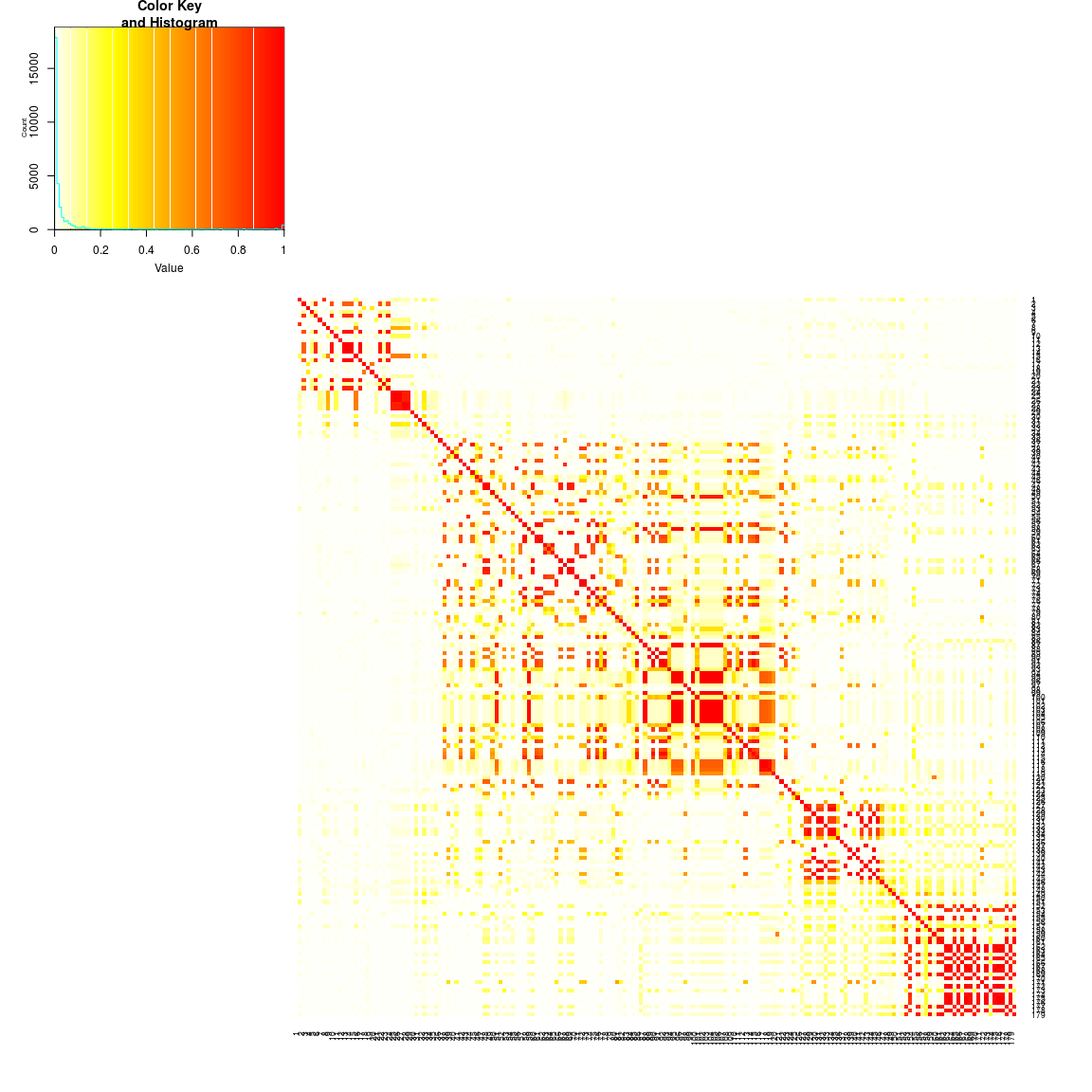## Introduction

sim1000G allows for easy simulation of unrelated individuals starting from sequencing 1000 genomes varians.

The following, somewhat complicated example, showcases the following:

• how to extract regions from 1000 genomes
• simulate individuals from the CEU+TSI+GBR individuals.
• simulate individuals from the ASW individuals.
• combinte the two simulated data into one dataset.
• perform SKAT test for the region and examine the effects of population stratification

The original 1000 genomes VCF files are obtained from 1000 genomes ftp site, at the location:

http://hgdownload.cse.ucsc.edu/gbdb/hg19/1000Genomes/phase3/

### Generating IDs of individuals to extract

sink(tempfile())
ped_file_1000genomes = system.file("examples", "20130606_g1k.ped", package = "sim1000G")

ped = read.table(ped_file_1000genomes,h=T,as=T,sep="\t")

pop1 = c("CEU","TSI","GBR")
id1 = ped$Individual.ID [ ped$Population %in% pop1 ]

id2 = ped$Individual.ID [ ped$Population == "ASW" ]

pop_map = ped$Population names(pop_map) = ped$Individual.ID

write_sample_files = 0

if(write_sample_files == 1) {
cat(c(id1,id2),file="/tmp/samples1.txt",sep="\n")
# cat(c(id2),file="/tmp/samples2.txt",sep="\n")
}

### Extracting variant sets using bcftools

We extract the CEU,TSI,GBR and ASW samples from a region of chromosome 4 from 73MBp to 74MBp using bcftools. The following command are run in the shell:


#77356278-77703432

INPUT_VCF=ALL.chr4.phase3_shapeit2_mvncall_integrated_v5a.20130502.genotypes.vcf.gz

bcftools view -S /tmp/samples1.txt -r 4:73000000-74000000 --force-samples $INPUT_VCF > /tmp/chr4-80.vcf bcftools filter -i 'AF>0 && EUR_AF>0 && AFR_AF>0' < /tmp/chr4-80.vcf | gzip > /tmp/chr4-80-filt.vcf.gz ### Reading the vcf file library(sim1000G) vcf_file = "/tmp/chr4-80-filt.vcf.gz" if(1) { examples_dir = system.file("examples", package = "sim1000G") vcf_file = file.path(examples_dir, "region-chr4-93-TMEM156.vcf.gz" ) } vcf = readVCF(vcf_file, maxNumberOfVariants = 200 , min_maf = 0.02, max_maf = 0.32) table( pop_map[ vcf$individual_ids ])

C = cor(t( vcf$gt1+vcf$gt2))^2
gplots::heatmap.2(C,col=rev( heat.colors(100) ) ,Rowv=F,Colv=F,trace="none",breaks=seq(0,1,l=101))#gplots::heatmap.2(cor(t(vcf2$gt1))^2,col=rev( heat.colors(100) ) ,Rowv=F,Colv=F,trace="none") if(0) { ids = vcf$individual_ids

id_pop1 = which(ids %in% id1)
id_pop2 = which(ids %in% id2)

gplots::heatmap.2(cor(t( vcf$gt1[,id_pop1]+vcf$gt2[,id_pop1]))^2,col=rev( heat.colors(100) ) ,Rowv=F,Colv=F,trace="none",breaks=seq(0,1,l=101))

gplots::heatmap.2(cor(t( vcf$gt1[,id_pop2]+vcf$gt2[,id_pop2]))^2,col=rev( heat.colors(100) ) ,Rowv=F,Colv=F,trace="none",breaks=seq(0,1,l=101))

}

### Starting two distinct simulations for the two sample sets and simulating unrelated individuals

#

startSimulation(vcf, subset = id1)

saveSimulation("pop1")

N = 200
id = c()
for(i in 1:N) id[i] = SIM$addUnrelatedIndividual() genotypes = retrieveGenotypes(id) gplots::heatmap.2(cor( genotypes )^2,col=rev( heat.colors(100) ) ,Rowv=F,Colv=F,trace="none",breaks=seq(0,1,l=101)) startSimulation(vcf, subset = id2) saveSimulation("pop2") N = 200 id = c() for(i in 1:N) id[i] = SIM$addUnrelatedIndividual()

genotypes2 = retrieveGenotypes(id)

gplots::heatmap.2(cor( genotypes2 )^2,col=rev( heat.colors(100) ) ,Rowv=F,Colv=F,trace="none",breaks=seq(0,1,l=101))

### Combining the two datasets and running SKAT

library(SKAT)

loadSimulation("pop1")

plot(apply(genotypes,2,mean), apply(genotypes2,2,mean))

gt = rbind(genotypes,genotypes2)

#gt = genotypes

dim(gt)

maf = apply(gt,2,mean,na.rm=T)/2
apply(gt,2,function(x) sum(is.na(x)))

flip  = which(maf > 0.5) ; gt[,flip] = 2 - gt[,flip]

#gt = genotypes

dim(gt)

maf = apply(gt,2,mean,na.rm=T)/2
plot(maf)

sum(maf==0)

apply(gt,2,function(x) sum(is.na(x)))

flip  = which(maf > 0.5)
gt[,flip] = 2 - gt[,flip]

dim(gt)

effect_sizes = rep(0, ncol(gt))
nvar = length(effect_sizes)

s = sample(1:nvar, 33)
effect_sizes[s] = 5

apply(gt[,s],1,sum)

predictor2 = function(b, geno) {
x = b
for(i in 1:ncol(geno)) { x = x  + b[i+1] * ( geno[,i] > 0) + b[i+1] * ( geno[,i] > 1)   }
exp(x) / (1+exp(x) )
}

p =predictor2 (  c(-1.5,effect_sizes) ,  gt)

phenotype = rbinom( length(p) , 1 , p )

#phenotype = sample(phenotype)
obj<-SKAT_Null_Model(phenotype ~ 1, out_type="D")

library(SKAT)
SKATBinary((gt),obj)\$p.value

#### Genetic maps in sim1000G

Through the functions readGeneticMap and downloadGeneticMap, we provide the functionality to automatically download genetic maps for GRCH37 build of the human genome.